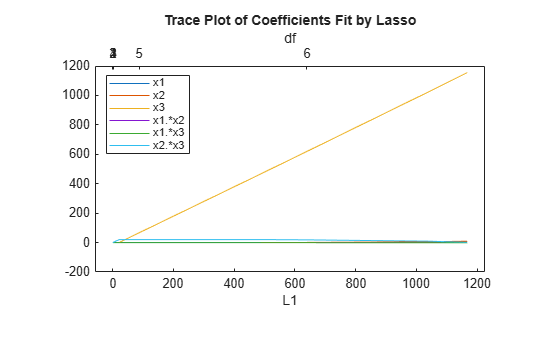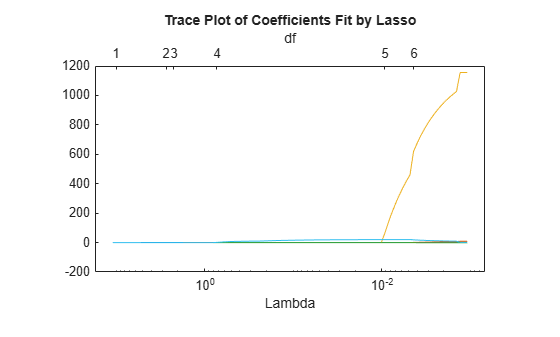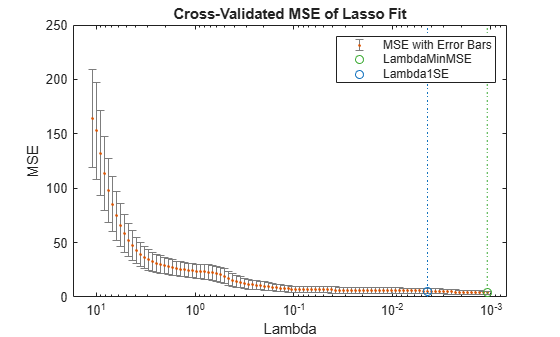Documentation

# lassoPlot

Trace plot of lasso fit

## Syntax

```lassoPlot(B) lassoPlot(B,FitInfo) lassoPlot(B,FitInfo,Name,Value) [ax,figh] = lassoPlot(___) ```

## Description

`lassoPlot(B)` creates a trace plot of the values in `B` against the L1 norm of `B`.

`lassoPlot(B,FitInfo)` creates a plot with type depending on the data type of `FitInfo` and the value, if any, of the `PlotType` name-value pair.

`lassoPlot(B,FitInfo,Name,Value)` creates a plot with additional options specified by one or more `Name,Value` pair arguments.

```[ax,figh] = lassoPlot(___)```, for any previous input syntax, returns a handle `ax` to the plot axis, and a handle `figh` to the figure window.

## Input Arguments

 `B` Coefficients of a sequence of regression fits, as returned from the `lasso` or `lassoglm` functions. `B` is a `p`-by-`NLambda` matrix, where `p` is the number of predictors, and each column of `B` is a set of coefficients `lasso` calculates using one `Lambda` penalty value. `FitInfo` Information controlling the plot: `FitInfo` is a structure, especially as returned from `lasso` or `lassoglm` — `lassoPlot` creates a plot based on the `PlotType` name-value pair.`FitInfo` is a vector — `lassoPlot` forms the x-axis of the plot from the values in `FitInfo`. The length of `FitInfo` must equal the number of columns of `B`.

### Name-Value Pair Arguments

Specify optional comma-separated pairs of `Name,Value` arguments. `Name` is the argument name and `Value` is the corresponding value. `Name` must appear inside quotes. You can specify several name and value pair arguments in any order as `Name1,Value1,...,NameN,ValueN`.

`'Parent'`

Axis in which to draw the plot.

Default: New plot

`'PlotType'`

Plot type when you specify a `FitInfo` vector or structure:

PlotTypePlot
`'L1'``lassoPlot` creates the x-axis from the L1 norm of the coefficients in `B`. The x-axis at the top of the plot contains the degrees of freedom (`df`), meaning the number of nonzero coefficients of `B`.

`'Lambda'`

When you choose this value, `FitInfo` must be a structure.

`lassoPlot` creates the x-axis from the `Lambda` field of `FitInfo`. The x-axis at the top of the plot contains the degrees of freedom (`df`), meaning the number of nonzero coefficients of `B`.

`'CV'`

When you choose this value, `FitInfo` must be a cross-validated structure.

• For each `Lambda`, `lassoPlot` plots an estimate of the mean squared prediction error on new data for the model fitted by `lasso` with that value of `Lambda`.

• `lassoPlot` plots error bars for the estimates.

If you include a cross-validated `FitInfo` structure, `lassoPlot` also indicates two specific `Lambda` values with green and blue dashed lines.

• A green, dashed line indicates the value of `Lambda` with a minimum cross-validated mean squared error (MSE).

• A blue, dashed line indicates the greatest `Lambda` that is within one standard error of the minimum MSE. This Lambda value makes the sparsest model with relatively low MSE.

To display the label for each plot in the legend of the figure, type `legend('show')` in the Command Window.

Default: `'L1'`

`'PredictorNames'`

String array or cell array of character vectors to label each coefficient of `B`. If the length of `PredictorNames` is less than the number of rows of `B`, the remaining labels are padded with default values.

`lassoPlot` uses `PredictorNames` in `FitInfo` only if:

• You created `FitInfo` with a call to `lasso` that included a `PredictorNames` name-value pair.

• You call `lassoPlot` without a `PredictorNames` name-value pair.

• You include `FitInfo` in your `lassoPlot` call.

For an example, see Lasso Plot with Default Plot Type.

Default: `{'B1','B2',...}`

`'XScale'`

• `'linear'` for linear x-axis

• `'log'` for logarithmic scaled x-axis

Default: `'linear'`, except `'log'` for the `'CV'` plot type

## Output Arguments

 `ax` Handle to the axis of the plot (see Axes Appearance (MATLAB)). `figh` Handle to the figure window (see Special Object Identifiers (MATLAB)).

## Examples

collapse all

`load acetylene`

Prepare the design matrix for lasso fit with interactions.

```X = [x1 x2 x3]; D = x2fx(X,'interaction'); D(:,1) = []; % No constant term```

The `x2fx` function returns the quadratic model in the order of a constant term, linear terms and interaction terms: constant term, `x1`, `x2`, `x3`, `x1.*x2`, `x1.*x3`, and `x2.*x3`

Fit a regularized model of the data using `lasso`.

`B = lasso(D,y);`

Plot the lasso fits with labeled coefficients by using the `PredictorNames` name-value pair.

```lassoPlot(B,'PredictorNames',{'x1','x2','x3','x1.*x2','x1.*x3','x2.*x3'}); legend('show','Location','NorthWest') % Show legend```Each line represents a trace of the values in B for a single predictor variable: `x1`, `x2`, `x3`, `x1.*x2`, `x1.*x3`, and `x2.*x3`.

Display a data tip for the trace plot. A data tip appears when you hover over a data tip.A data tip displays these lines of information: the name of the selected coefficient with a fitted value, the L1 norm of a set of coefficients including the selected coefficient, and the index of the corresponding Lambda.

`load acetylene`

Prepare the data for lasso fit with interactions.

```X = [x1 x2 x3]; D = x2fx(X,'interaction'); D(:,1) = []; % No constant term```

Fit a regularized model of the data with `lasso`.

`[B,FitInfo] = lasso(D,y);`

Plot the fits with the `Lambda` plot type and logarithmic scaling.

`lassoPlot(B,FitInfo,'PlotType','Lambda','XScale','log');`Visually examine the cross-validated error of various levels of regularization.

`load acetylene`

Create a design matrix with interactions and no constant term.

```X = [x1 x2 x3]; D = x2fx(X,'interaction'); D(:,1) = []; % No constant term```

Construct the lasso fit using 10-fold cross-validation. Include the `FitInfo` output so you can plot the result.

```rng default % For reproducibility [B,FitInfo] = lasso(D,y,'CV',10);```

Plot the cross-validated fits.

```lassoPlot(B,FitInfo,'PlotType','CV'); legend('show') % Show legend```The green circle and dotted line locate the `Lambda` with minimum cross-validation error. The blue circle and dotted line locate the point with minimum cross-validation error plus one standard deviation.